# 7+ Division Worksheet Examples in PDF

Along with Multiplication, Division is also one of the basic math operations or arithmetic functions that we make use of in our day-to-day lives. However, it is said to be the most difficult arithmetic functions because, like multiplication, division is a slow operation. But nowadays multiplication can now be solved way faster than division but the fact remains that addition and subtraction are the easier ones.## What is Division?

Division is basically the process of taking something from a whole item into equal groups. When you divide, you have no choice but to start with a large number before breaking it into small but equal pieces depending on the number of your divisor. You may also like grammar worksheets.

### Division Terminologies

Now, division, like the other basic operations in math, has its own special terms when it comes to its parts in an equation. Take a look at this equation: 10 ÷ 2 = 5. The number 10 here is the dividend– it is the number that is being divided. The number 2 here is the divisor and it is the number that we use in identifying how many groups from the dividend, which is number 10, that we are going to make. The answer you can come up with after dividing is called the quotient, so in the given equation, number 5 is the quotient. You may also like biography worksheet examples.

When you would divide a number and that you would get a whole number, it means it’s divisible. For example, when you would divide 10 by 2, the answer is 5. Five is a whole number which also means that 5 is divisible by 10. You may also check out coaching worksheet examples in pdf.

If you would divide numbers and there is a so-called leftover number, it is called as a “remainder”. For example, when you would divide 7 by 2, the quotient is 3 and the remainder is number 1. This is the time when you would borrow and drop down a zero. You might be interested in goal setting worksheets.

### Steps in Division

When we divide, we actually make use of the other basic operations. When we divide, the order goes like this:

1. Divide
2. Multiply
3. Subtract
4. Drop down

Unless you come up with a final answer, you would have to repeat these steps until you come up with the final answer.

## Division Worksheet Example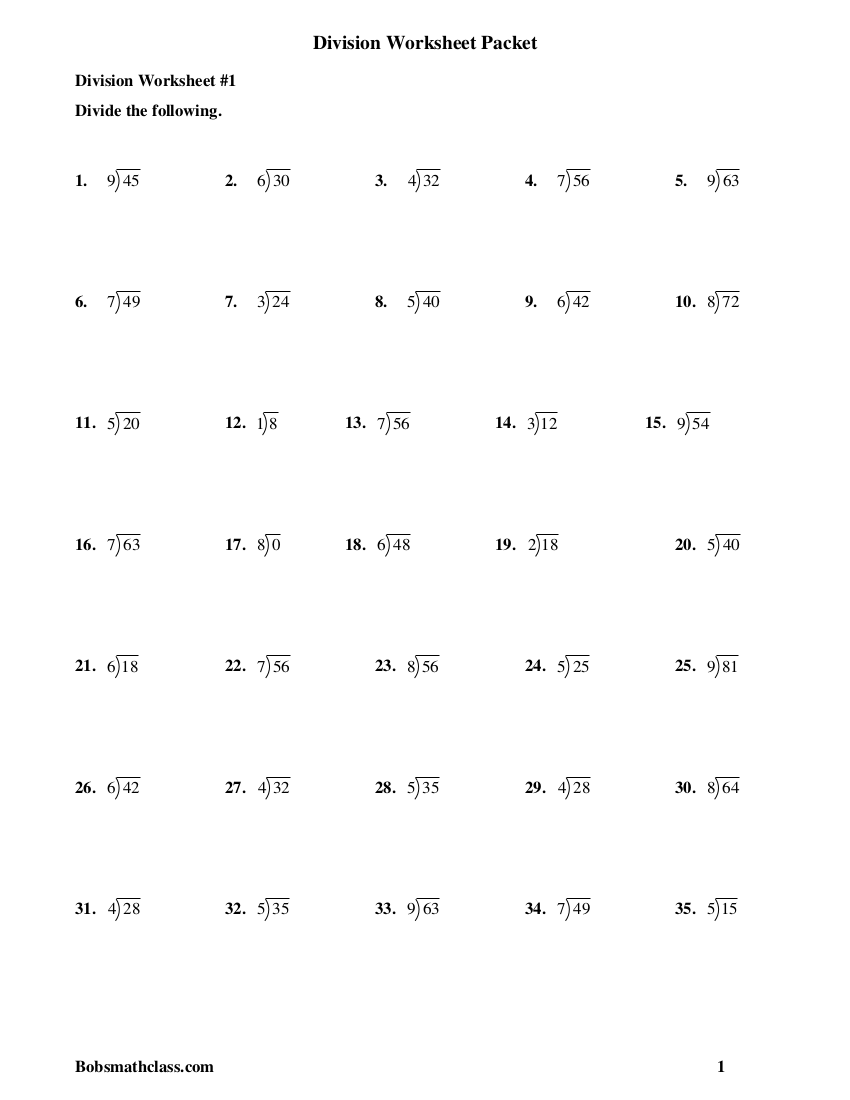bobsmathclass.co

## Division Grade 4 Practice Worksheet Example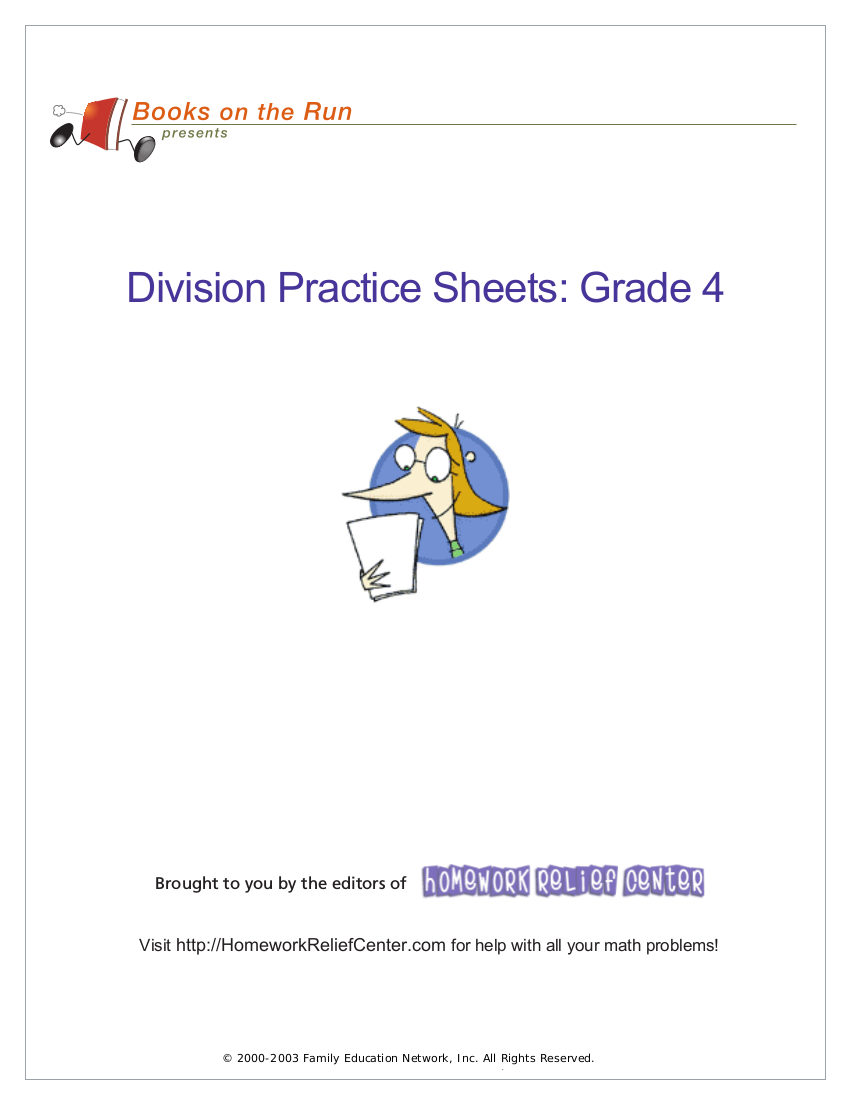steveshapero.com

## Division Made Easy Worksheet Example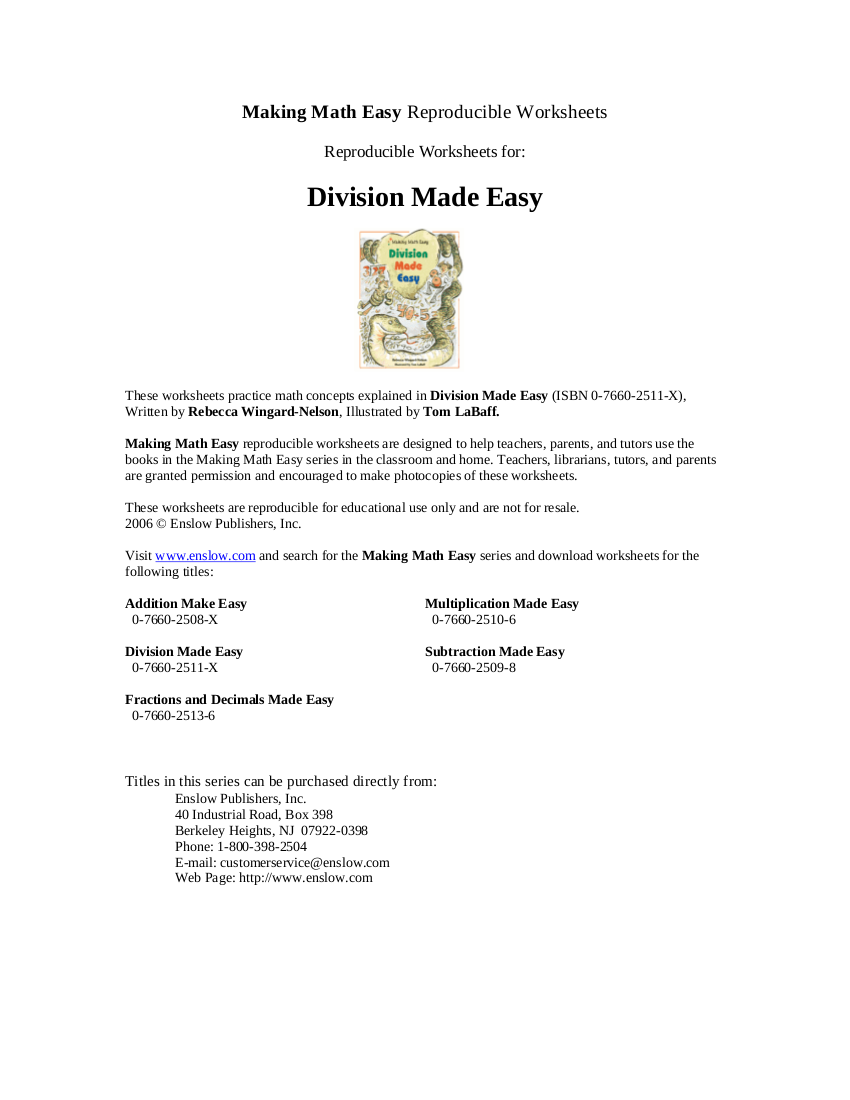mathematicshed.co

## Three-minute Math Division Worksheet Example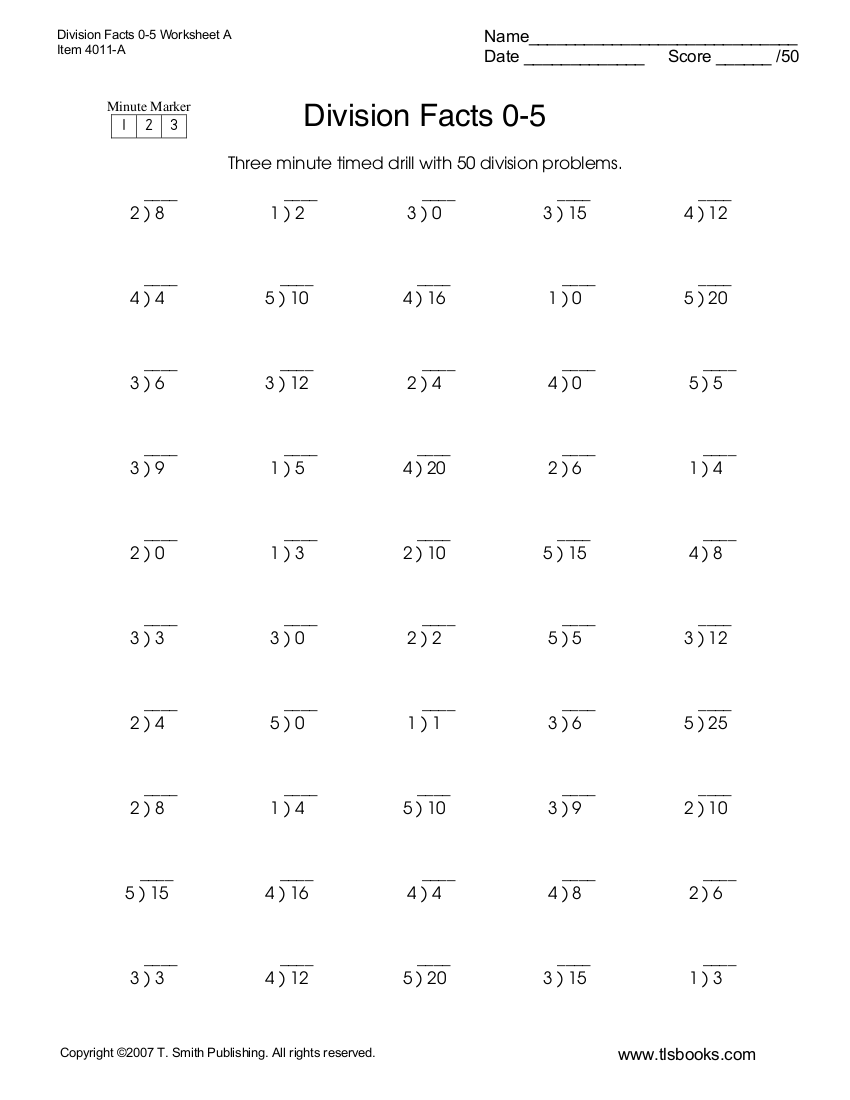tlsbooks.com

## Quick Rules on Division

• When we divide using paper or on a spreadsheet, the sign we use for division is (÷). But on a computer or on our cellular phones, we would make use of the ‘/’ symbol in order to denote division.
• If division is the inverse version for multiplication, the same goes for the multiplication as the opposite of multiplication in mathematics.
• Are you fully aware that when you divide, you are actually doing a quick way of doing multiple additions and a quick way of doing multiple subtractions?
• Division is actually considered as the hardest of the four main arithmetic functions, which includes addition, subtraction, and multiplication.
• Just like multiplication, anything that you divide with 0, the answer will also be zero, for example:
• 0 ÷ 1 = 0
• 0 ÷ 2 = 0
• 0 ÷ 3 = 0
• 0 ÷ 4 = 0
• 0 ÷ 5 = 0
• 0 ÷ 6 = 0
• 0 ÷ 7 = 0
• 0 ÷ 8 = 0
• 0 ÷ 9 = 0
• 0 ÷ 10 = 0
• In short, when you are going to divide any number with 0, you are simply not dividing at all. And this fact often causes quite a problem in mathematics because come to think of it: You are given a problem that states you are going divide 8 with 0, and if you would apply it in real-life setting, let’s say divide 8 slices of pizza to nobody, you are not exactly dividing the 8 slices of pizza. Get it? You cannot simply divide by 0.
• Now aside from the puzzling case of dividing any number with 0, there is also the case of the number 1. When you would be dividing 1 with any number, the answer will always be the same as the number you divided number 1 with. For example:
• 1 ÷ 1 = 1
• 2 ÷ 1 = 2
• 3 ÷ 1 = 3
• 4 ÷ 1 = 4
• 5 ÷ 1 = 5
• 6 ÷ 1 = 6
• 7 ÷ 1 = 7
• 8 ÷ 1 = 8
• 9 ÷ 1 = 9
• 10 ÷ 1 = 10
• Here’s another case of the number 1: when you would divide two similar numbers, it would always end up with the number 1. For example
• 1 ÷ 1 = 1
• 2 ÷ 2 = 1
• 3 ÷ 3 = 1
• 4 ÷ 4 = 1
• 5 ÷ 5 = 1
• 6 ÷ 6 = 1
• 7 ÷ 7 = 1
• 8 ÷ 8 = 1
• 9 ÷ 9 = 1
• 10 ÷ 10 = 1
• Unlike the rest of the basic operations addition, subtraction, and multiplication, you should always divide with a correct order. If you interchange the number, the answer will be a different number. For example, when you divide 8 by 2, the answer is 4 whereas when you divide 2 by 8, the answer will be 0.25. The former is like dividing 8 slices of pizza for two people while the latter is like dividing 2 slices of pizza to 8 people.

## Long Division Worksheet Example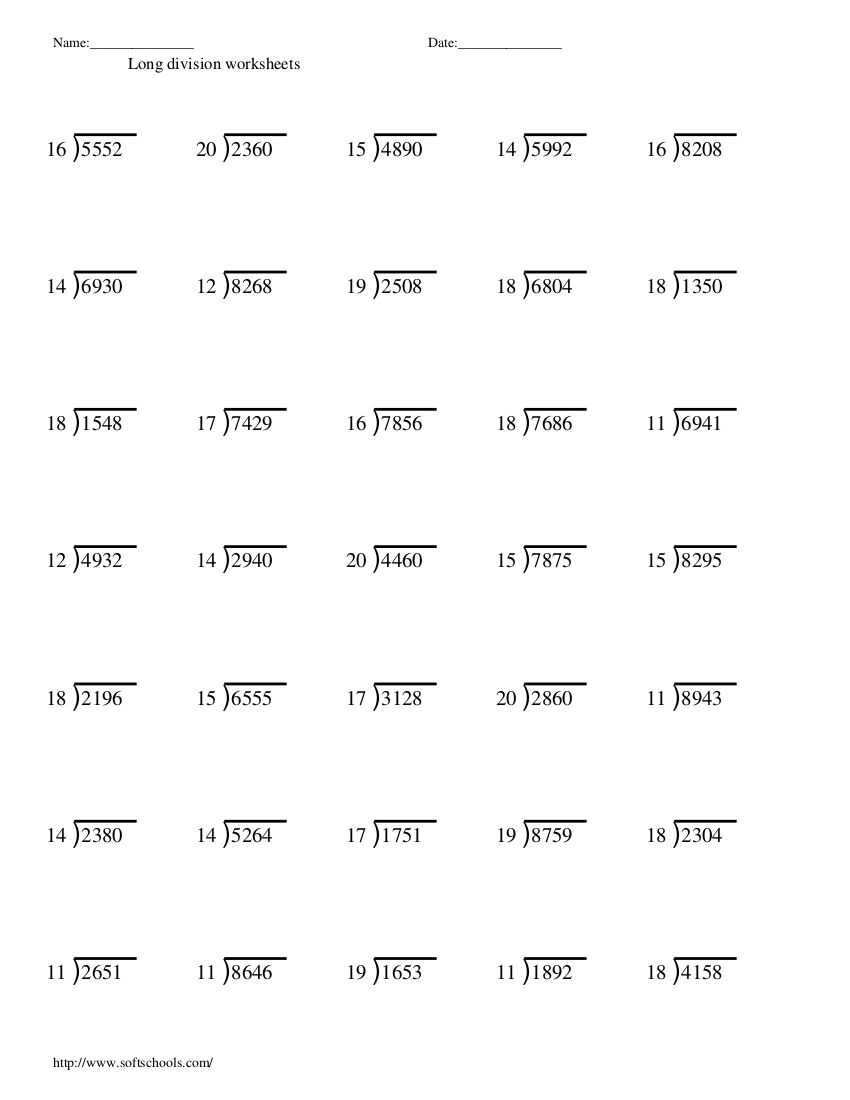softschools.com

## Timed Division Drill Worksheet Example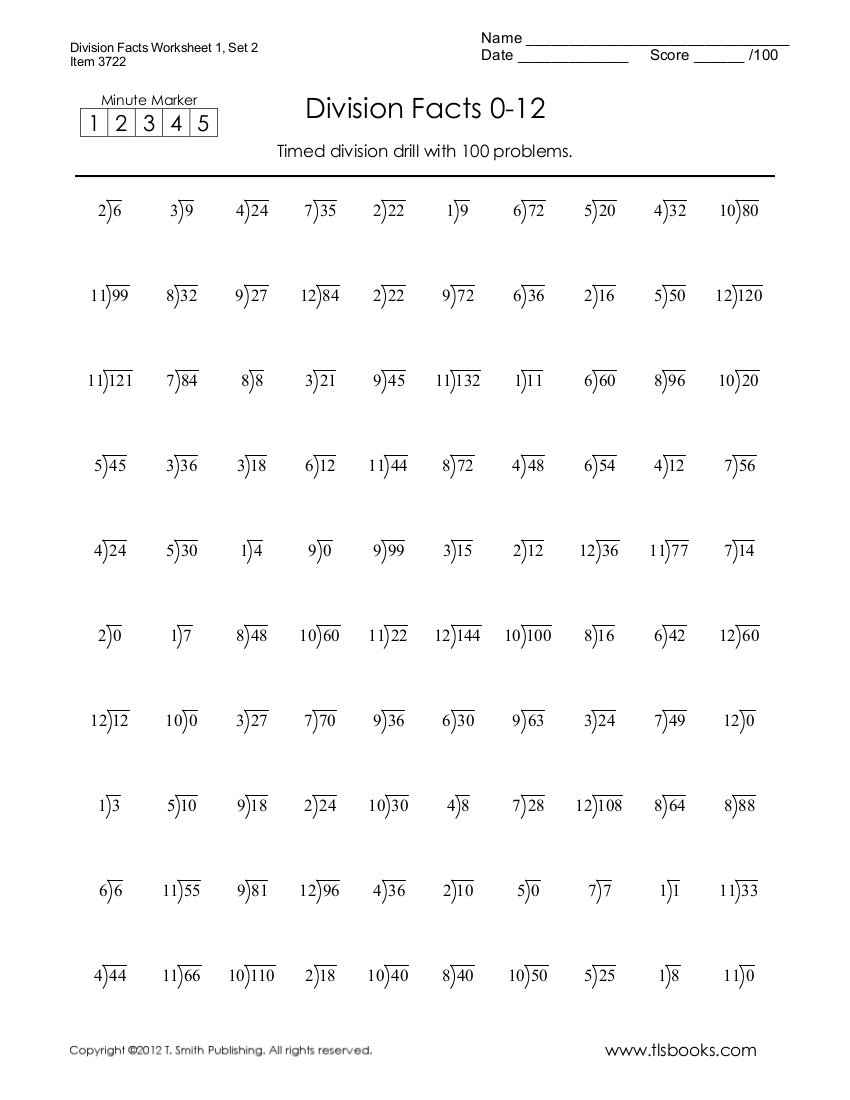teachers.stjohns.k12.fl.us

## Multiplying and Dividing Fractions Worksheet Example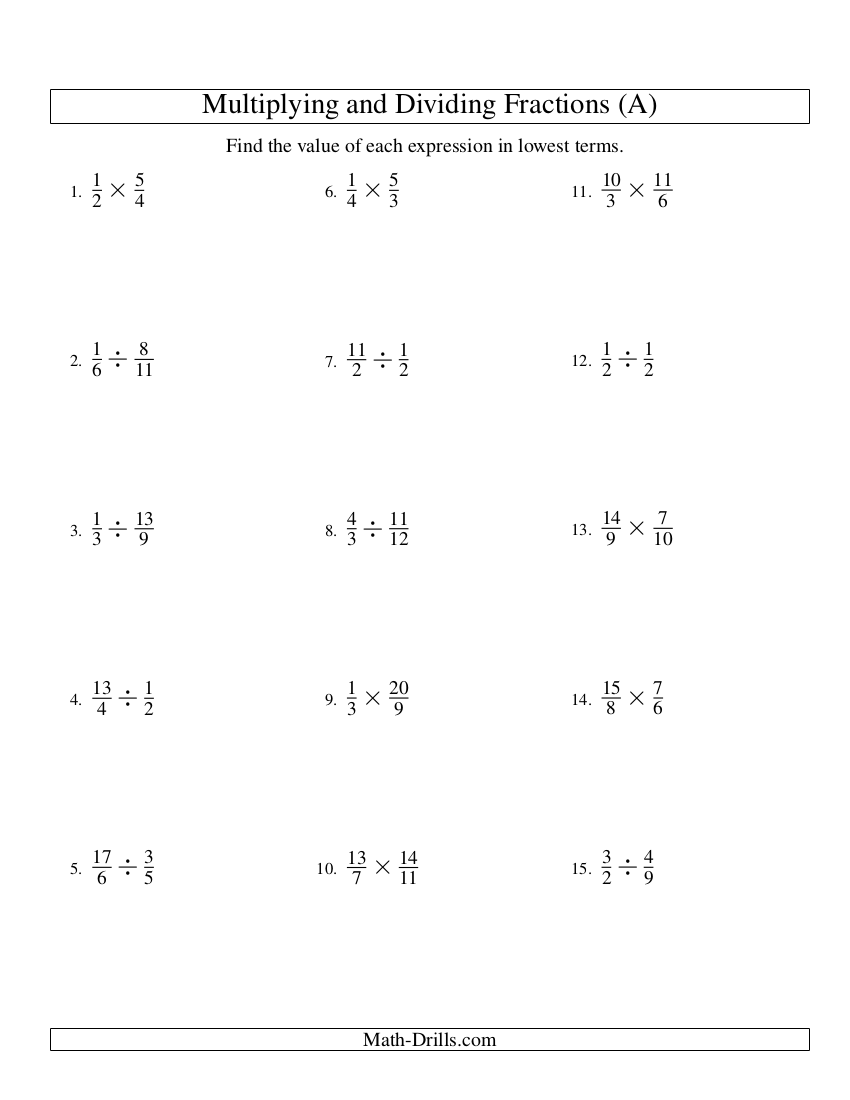clatsopcc.edu

## Dividing Polynomials Worksheet Example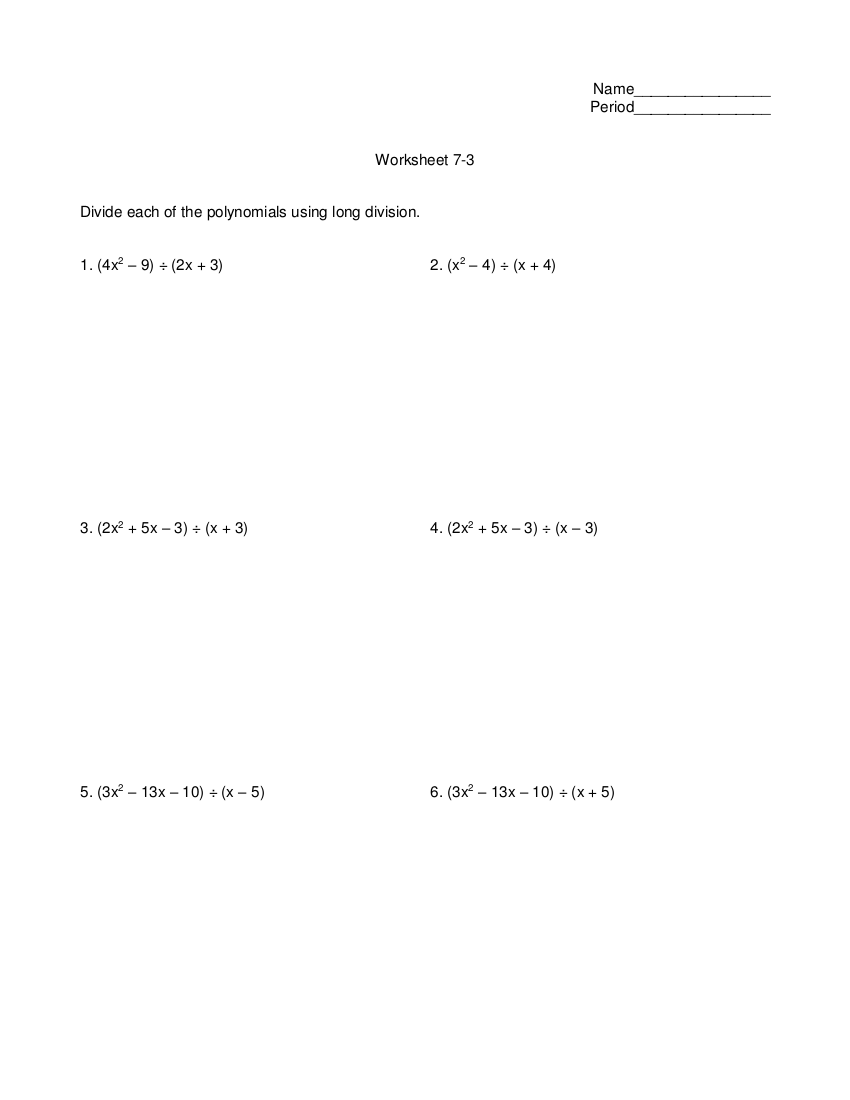tamdistrict.or

## Division and Some of its Real-Life Applications

Math is more than the numbers that we see; it’s a part of our lives. Can you even imagine a world without math? You simply cannot do so. You may also check out expense worksheet examples in pdf.

We may not be aware of it but we have been using math even in the absence of numbers. We have been dividing things, not knowing that we are already making use of the operation in our daily activities. You may also like resume worksheet examples in pdf.

Should you want to know some of the ways how the operation of division is used in our lives, here are some of the ways that we have been making use of this arithmetic function:

### Organizations

Do you have an upcoming event? Whatever kind of event it might be, an event is something that involves of tasks that should be divided by the members of the organizing body of the event. For example, your organization is about to hold an event. Now, if you would apply division, you would then have to divide the tasks necessary for the project to be completed. Such tasks include the logistics department, the operations, promotions, and if you are planning to provide giveaways, you might need merchandising. For you to effectively carry out the event effectively, you should not own all of the tasks and you must delegate it to others. You may also check out math worksheets for students.

However, the tendency of organizing an event is that there is always a possibility wherein, due to all the stress and problems, the organizing body will be experiencing division among its members. It is normal that the people in an organization would have varying opinions and this can definitely cause problems and division among the members. But if the members would choose a path wherein they will not be divided with their personal opinions and unadvised decisions, division among the members will less likely to happen or when it does, it would not cause too many problems that will eventually lead to the canceling of the upcoming event. You may also see activity sheet examples & samples.

### Within Family Context

Imagine big families and that their only source of income is from their father. The mother does all the budgeting in order to make sure that they would make the ends meet without any worries. The process of budgeting is an act of dividing. When you budget, you have to divide your income into several categories. You may also like self-assessment worksheet examples in pdf.

It is also in budgeting where you would apply the operation. But more than that, if you would look at it in the eyes of the mother or the one who budgets the finance of a big family, budgeting and dividing the scanty income of the family’s sole breadwinner is the most difficult challenge in their lives to the point that they would be sacrificing their own share just so the other, particularly the younger ones, to have more share. You may also see sheet examples in DOC.

Another real-life application of division within family context is the role of the family members. The father typically gets the role as a breadwinner, while the mother takes on the role as a housewife. There is also a division of tasks among siblings and such division of tasks includes who cleans or sweeps, who cooks, who washes the dishes, who does the laundry, who folds the freshly washed clothes, who takes care of the surroundings of their homes, among others. You might be interested in reading comprehension worksheet examples in PDF.

We hope you could make use of these division worksheets we have provided for you in this article. You may also see Math Worksheets for Students.